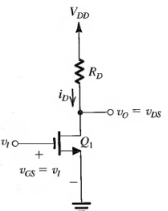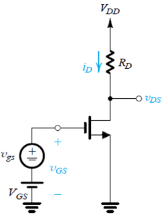# Test: MOSFET In Amplifier Design

## 10 Questions MCQ Test Electronic Devices | Test: MOSFET In Amplifier Design

Description
This mock test of Test: MOSFET In Amplifier Design for Electrical Engineering (EE) helps you for every Electrical Engineering (EE) entrance exam. This contains 10 Multiple Choice Questions for Electrical Engineering (EE) Test: MOSFET In Amplifier Design (mcq) to study with solutions a complete question bank. The solved questions answers in this Test: MOSFET In Amplifier Design quiz give you a good mix of easy questions and tough questions. Electrical Engineering (EE) students definitely take this Test: MOSFET In Amplifier Design exercise for a better result in the exam. You can find other Test: MOSFET In Amplifier Design extra questions, long questions & short questions for Electrical Engineering (EE) on EduRev as well by searching above.
QUESTION: 1

Solution:
QUESTION: 2

Solution:
QUESTION: 3

### (Q.3-Q.5) Consider the amplifier below for the case VDD = 5 V, RD = 24 kΩ, (W/L) = 1 mA/V2, and Vt = 1 V.If the amplifier is biased to operate with an overdrive voltage VOV of 0.5 V, find the incremental gain at the bias point.

Solution:
QUESTION: 4

For amplifier biased to operate with an overdrive voltage of 0.5V, and disregarding the distortion caused by the MOSFET’s square-law characteristic, what is the largest amplitude of a sine-wave voltage signal that can be applied at the input while the transistor remains in saturation?

Solution:

Use the standard mathematical formula to obtain the result.

QUESTION: 5

For the input signal of 1.5V what is the value of the gain value obtained?

Solution:

The amplitude of the output voltage signal that results is approximately equal to Voq – VOB = 2 – 0.61 = 1.39v.
The gain implied by amplitude is
Gain = -1.39/0.11 = -12.64 V/V.

QUESTION: 6

Which of the following is the fastest switching device?

Solution:

MOSFET is the fastest switching device among the given four options.

QUESTION: 7

Bias point is also referred by the name

Solution:

Bias point is called dc operating point as the MOSFET functions best at this point. Also since at the bias point no signal component is present it is called quiescent point (he reason why it is represented by the symbol ‘Q’)

QUESTION: 8

(Q.8 –Q.10) Consider the amplifier circuit shown below. The transistor is specified to have Vt = 0.4 V, kn = 0.4 mA/V2, W/L = 10 and λ = 0. Also, let VDD = 1.8V, RD = 17.5kΩ, VGS = 0.6V and vgs = 0V.Q. Find ID.

Solution:
QUESTION: 9

Find VDS.

Solution:
QUESTION: 10

Find Av.

Solution:

Av = – kn Vov RD
= -0.4 * 10 * 0.2 * 17.5
= – 14.4v